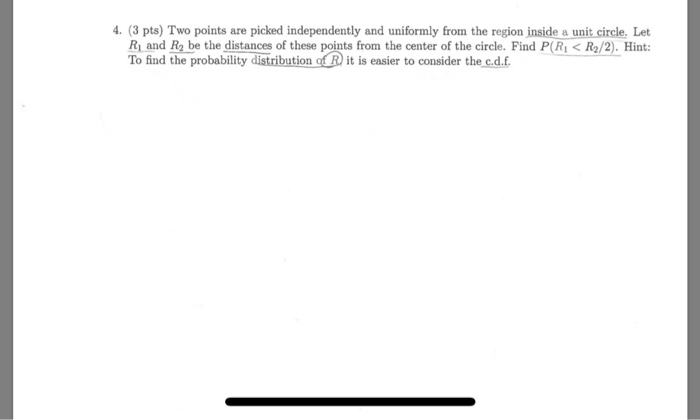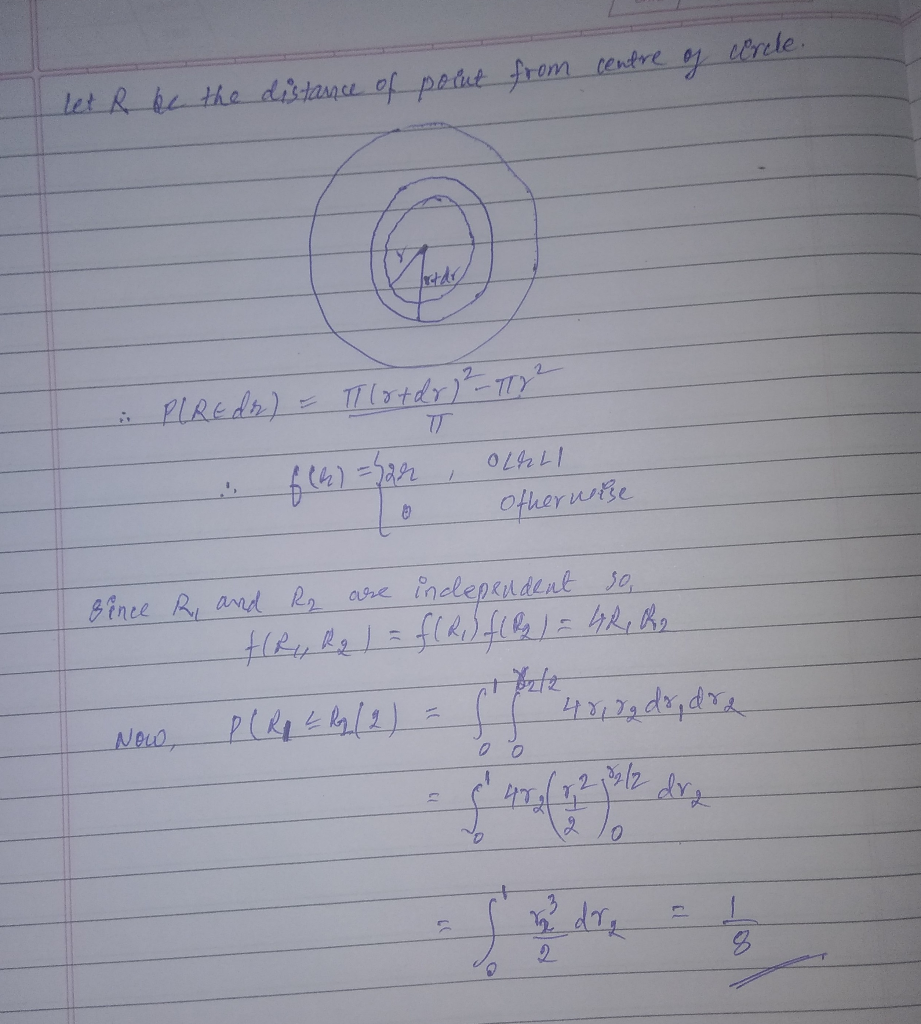# 4. (3 pts) Two points are picked independently and uniformly from the region inside a unit circle...4. (3 pts) Two points are picked independently and uniformly from the region inside a unit circle. Let R1 and R2 be the distances of these points from the center of the circle. Find P(RIR2/2). Hint: To find the probability distribution oR it is easier to consider the c.d.f.##### Add Answer of: 4. (3 pts) Two points are picked independently and uniformly from the region inside a unit circle...
More Homework Help Questions Additional questions in this topic.

• #### If a dart is thrown at a square target with a circle inscribed in it such that each side of the square is tangent to the circle, what is the approximate probability that the ball will hit inside the circle

Need Online Homework Help?